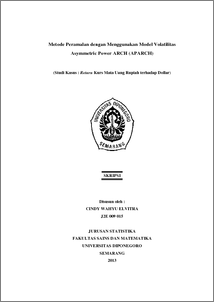# Metode Peramalan dengan Menggunakan Model Volatilitas Asymmetric Power ARCH (APARCH) (Studi Kasus : Return Kurs Mata Uang Rupiah terhadap Dollar)

ELVITRA, CINDY WAHYU (2013) Metode Peramalan dengan Menggunakan Model Volatilitas Asymmetric Power ARCH (APARCH) (Studi Kasus : Return Kurs Mata Uang Rupiah terhadap Dollar). Undergraduate thesis, UNIVERSITAS DIPONEGORO.Preview
PDF
886Kb

## Abstract

Exchange rate can be defined as a ratio the value of currency. The exchange rate shows a currency price, if it exchanged with another currency. Exchange rates of a currency fluctuate all the time. Rise and fall exchange rates of a currency in the money market shows the magnitude of volatility occurred in a country currency to other's. To estimate the volatility behavior of the data gave rise to volatility clustering or heteroscedasticity problems, can’t be modeled using ARMA model and asymmetric effects that can‘t be modeled by ARCH or GARCH, can be modeled by Asymmetric Power ARCH (APARCH). In determining the estimated parameter values of APARCH model, used the maximum likelihood method, followed by using the iteration method is Berndt, Hall, Hall and Hausman (BHHH). The APARCH model used to the data return of exchange rate against dollar is APARCH(2,1) or in the form as follows : σ_t^2,064874 = 0,00000268 + 0,830902 〖(|ɛ_(t-1) | + 0,138204 ɛ_(t-1))〗^(2,064874 )+ 0,130516 σ_(t-1)^2,064874 + 0,074784 σ_(t-2)^2,064874 + 0,151157 σ_(t-3)^2,064874 Keywords: Exchange Rate, Volatility Clustering, ARCH, Return, APARCH

Item Type: Thesis (Undergraduate) H Social Sciences > HA Statistics Faculty of Science and Mathematics > Department of Statistics 42519 Mr Hasbi Yasin 27 Feb 2014 09:18 27 Feb 2014 09:18

Repository Staff Only: item control page